# Basic & Derived SI Units

### Basic SI Units

#### SI unit

length
meter
mass
kilogram
time
second
current
ampere

 The basic unit of length in the SI system is the meter (m), about 10% longer than a yard. The basic unit of mass in the SI system is the kilogram (kg), which is about 10% bigger than two pounds. The basic unit of time in SI is the second (s). In SI, the basic electrical unit is the ampere (A). (The British system has no electrical units whatsoever.) There are a few other basic units in the SI system, but they are not needed for this discussion.

## Units in combination with other units

 There are SI units for many any and all useful physical quantities, of which just a few are given in the table below. Importantly, the SI system is a system. If you put SI values into a formula for all quantities, the answer always comes out in SI units.  On the Internet, it is not easy to express superscripts without using pictures that take too much time to load.  Therefore, we use a caret to express the exponent.  For example, we use m^2 to represent square meters.

 NAME DEFINITION UNIT Area length ´  width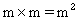volume length ´  width ´  height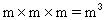velocity distance ¸ time m/s acceleration velocity change ¸ time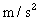force push or pull = mass ´  acceleration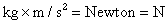energy capacity to do work W = force ´  distance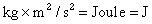power energy ¸ time J/s = watt = W intensity (solar) power ¸ area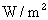It is important to remember that energy is measured in joules, and power is measured in watts. One joule = 1 watt ´ 1 second. People usually associate the watt with electricity, but that is because the SI system has traditionally been used only for electricity in the US. The watt is a general unit for power --- energy divided by time --- regardless of whether electricity is involved.  The so-called power company sends you a bill for energy (not power), but it uses a non-SI unit for time. To get the energy, they multiply kilowatts by hours to get kWh.

To main Energy Advocate IndexThe Energy Advocate